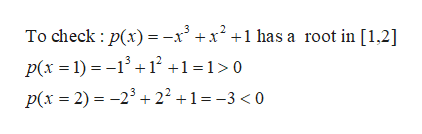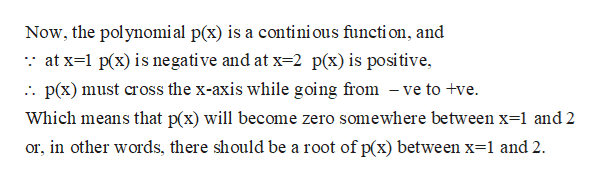# Show that the polynomial p(x) = -x^3+x^2+1 has a root in [1,2]

Question
1 views

Show that the polynomial p(x) = -x^3+x^2+1 has a root in [1,2]

check_circle

Step 1

We can check for the values of p(x) :help_outlineImage TranscriptioncloseTo check : p(x) = -x' +x² +1 has a root in [1,2] p(x =1) = -1° + 1² +1 =1>0 p(x = 2) = -23 +2² +1= -3 < 0 fullscreen
Step 2

Thus,

...help_outlineImage TranscriptioncloseNow, the polynomial p(x) is a contini ous functi on, and :: at x=1 p(x) is negative and at x=2 p(x)is positive, :. p(x) must cross the x-axis while going from – ve to +ve. Which means that p(x) will become zero somewhere between x=l and 2 or, in other words, there should be a root of p(x) between x=1 and 2. fullscreen

### Want to see the full answer?

See Solution

#### Want to see this answer and more?

Solutions are written by subject experts who are available 24/7. Questions are typically answered within 1 hour.*

See Solution
*Response times may vary by subject and question.
Tagged in

### Other